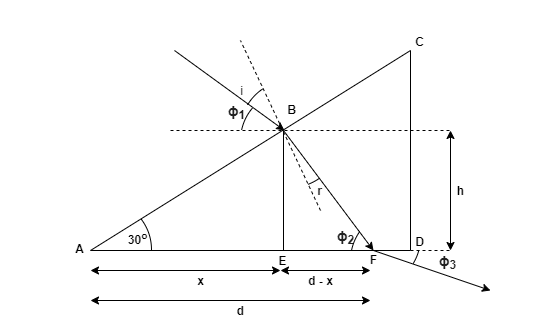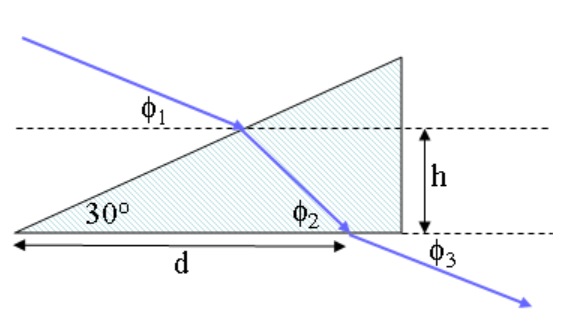# Problem: Red light is incident in air on a 30- 60- 90(degree) prism as shown. The incident beam is directed at an angle of ϕ1 = 41.3o with respect to the horizontal and enters the prism at a height h = 17 cm above the base. The beam leaves the prism to the air at a distance d = 45.5 along the base as shown.What is ϕ2, the angle the beam in the prism makes with the horizontal axis?

###### FREE Expert Solutiontan (30) = (h/x)

x = h/tan(30) = 17/tan(30) = 29.44 cm

88% (356 ratings)###### Problem Details

Red light is incident in air on a 30- 60- 90(degree) prism as shown. The incident beam is directed at an angle of ϕ1 = 41.3o with respect to the horizontal and enters the prism at a height h = 17 cm above the base. The beam leaves the prism to the air at a distance d = 45.5 along the base as shown.What is ϕ2, the angle the beam in the prism makes with the horizontal axis?